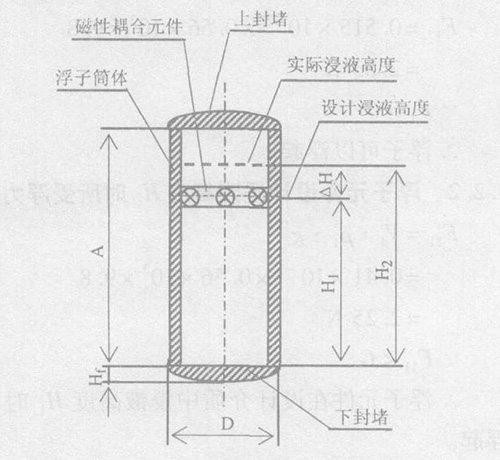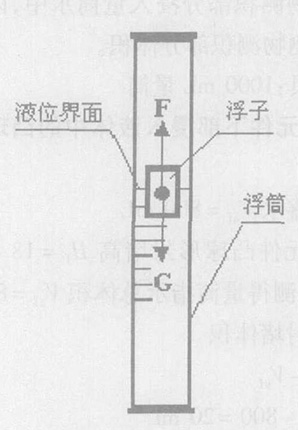服务热线：15195518515

# 侧装式磁翻板液位计在储罐液面测量中的选用及计算方法

1、前言

2000年11月, 某新建液化石油气站在维修不

2、侧装式磁翻板液位计的选用

2.1　浮子元件几何尺寸应符合储罐内盛装介质的密度要求2.2　浮子元件材料

V ρ g >m g

2.3　指示误差

3、对浮子元件的测量
3.1　浮子元件直径测量

2=51.0 +49.1
2=50.05 mm
3.2　浮子元件体积测量

3.2.1　浮子元件下部浸入液体中的凸球形封堵体积Vf量筒内盛水Vs f =800 mL将浮子元件凸球形封堵高Hf =18 mm 部分浸入量筒水中, 测得量筒指示总体积Vz f =820mL。凸球形封堵体积
Vf =Vz f -Vs f
=820 - 800 =20mL
=0.02 ×10- 3 m3
3.2.2　浮子元件设计标定浸入液体中高度为H1 时
(磁性元件安装于浮子内位置)的体积V1量筒内盛水Vs1 =500mL将浮子元件设计标定浸入液体中高度为H1 =200 mm以下段浸入量筒水中, 测得量筒指示总体积Vz1 =910mL。

V1 =Vz1 -Vs1
=910 -500 =410 mL
=0.41 ×10 -3m3
3.2.3　浮子元件全体积V

V =Vz2 -Vs2
=965 -450
=515mL =0.515 ×10- 3 m3
3.2.4　浮子元件质量测量

m =255.0 g =0.255 kg
4、浮子元件浮力及指示液位性能计算4.1　浮子元件在介质内所受重力
G =m g =0.255 ×9.8 =2.499 N
4.2　浮子元件按出厂标明状态的性能验算
4.2.1　浮子全浸入标明密度的介质中所受浮力其中, ρ1为标明介质密度。

F 1 =0.515 ×10-3 ×0.56 ×103 ×9.8=2.83 N
∵F1 >G
∴浮子可以浮起。
4.2.2　浮子元件设计浸液高度H1 时所受浮力验算
F 11 =V1 ρ1 g=0.41 ×10-3 ×0.56 ×103 ×9.8=2.25 N
∵F11 ∴浮子元件在设计介质中浸液高度H1 时不能浮起。若使浮子元件呈浮起状态, 就要增加浮子元件浸入液体体积, 增加浮力至F 12 =G, 此时实际浸液高度H2 >H1 , 则浮子元件存在指示误差。
4.2.3　浮子元件指示误差验算设:浮子元件在设计介质内实际浸液高度H2 处所受的重力与浮力达到平衡状态:
F 12 =G

V2 =m g
ρ1 g=m
ρ1= 0.255
0.56 ×103=0.455 ×10-3 m

4-π D2 H1
4= π D2
4(H2 -H1 )
ΔH =H2 -H1 =4 ΔV
π D2= 4 ×(0.455 ×10-3 -0.410 ×10-3 )
π ×(50.05 ×10- 3 )2=2.3 ×10- 2 m =2.3 cm

∵ΔH >ΔHs
∴浮子元件在设计介质中的实际浸液高度高于

F2 =V ρ2 g=0.515 ×10- 3 ×0.42 ×103 ×9.8=2.12 N
∵此时F2 >G
∴该浮子元件在液化石油气介质中不能浮起,

5、结论

1.液化气体储罐液面计如选用磁浮子式, 应注意液面计适用的介质密度, 如果是液化石油气介质, 应选用薄不锈钢板材料、体积稍大、重量较轻的浮子元件, 并一定在安装前检查确认浮子元件。
2. 环境温差较大的液化气体储罐选用液面计指示误差要尽可能小, 并要进行误差测量、验算。
3. 液化气体储罐进行定期检验时, 液面计应作为检验重点, 要对浮子元件进行检查、测量, 并进行浮子元件性能验算, 防止因浮子元件损坏或选择不当而导致液面计失效, 造成事故。
4. 浮子元件是中空薄壳结构, 要保证浸在液体中能够浮起指示液位, 就要在承受外压时具有良好的密封性能。在对浮子元件检查时要注意检查元件的焊缝及表面, 不得有裂纹、气孔等贯穿缺陷和导致元件渗漏、不能保证密封性能的缺陷。

© 2018 金湖凯铭仪表有限公司 版权所有   |   备案号：苏ICP备15042790号-3